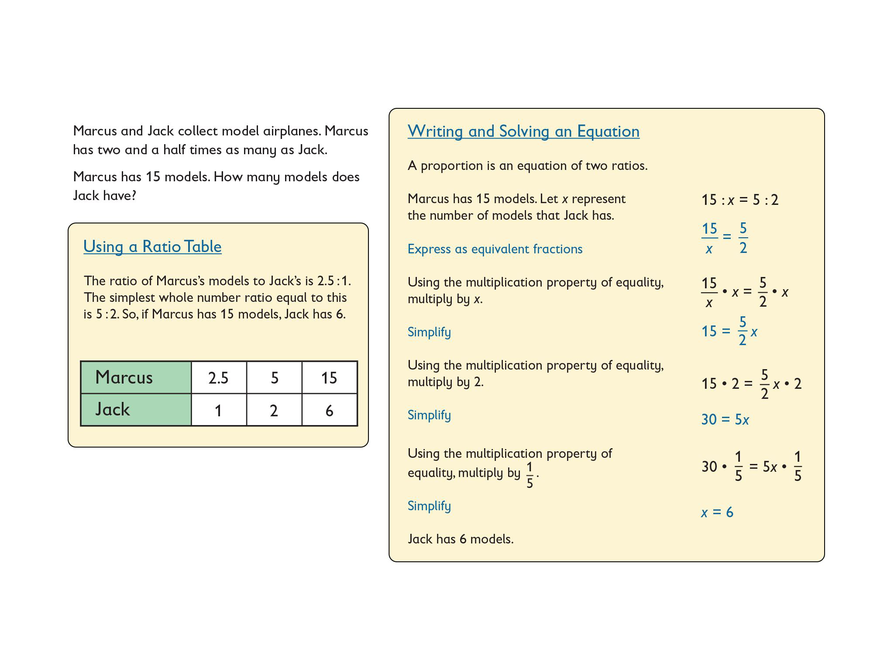# Multiplication Properties of Equality

## Opening

When working by yourself, you should:

• Make sense of the problem.
• Use “ask myself” questions to see what you need to understand before starting.
• Persevere through difficulty by using what you do know.

Read the worked problem. Then watch your teacher give an example of how to use “ask myself” questions to understand the problem.

• Solve the problem using a ratio table and using an equation.
• Describe how you used the multiplication property of equality to solve the equation.## Hint:

Here are ideas for “ask myself” questions:

• What does ____ mean?
• What is this problem talking about?
• What kinds of comparisons is the problem looking at?
• What are the numbers in the problem and what do they mean?
• What is the problem asking for?

Here are ideas for what to do if you get stuck:

• Look at similar problems you have solved previously.
• Model the problem using counters or other materials.
• Sketch a diagram or other representation.
• Write what you do know.
• Write down questions to ask later.
• Check other resources.
2 of 8